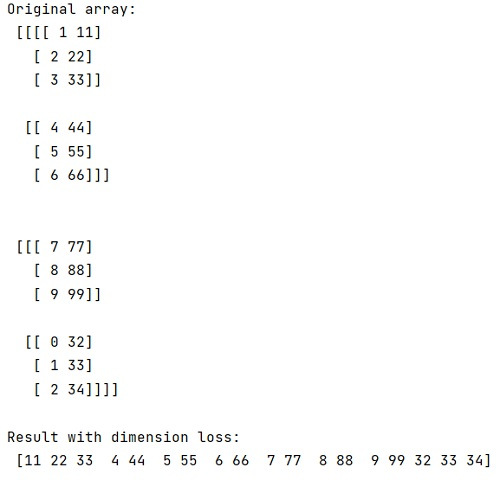# Loss of dimension during masking a NumPy Array (Solution)

In this tutorial, we will learn why there is loss of dimension when we mask a NumPy array, how to regain the original dimension? By Pranit Sharma Last updated : April 22, 2023

Suppose that we are given a multidimensional numpy array and we want to select certain elements of an array and perform a weighted average calculation based on the values. However, using a filter condition, destroys the original structure of the array arr which was of shape (2, 2, 3, 2) and is turned into a 1-dimensional array.

This is because normal filtering returns a flat array of only those values which satisfy the condition.

## How to regain the original dimensions?

To regain the original dimensions, you can use np.ma.masked_array() to represent the subset of elements that satisfy our condition. You can also access the data and the mask via the .data and .mask attributes respectively.

Let us understand with the help of an example,

## Python solution for losing of dimension during masking a NumPy Array

```# Import numpy
import numpy as np

# Creating an array
arr = np.asarray([[[[1, 11], [2, 22], [3, 33]],
[[4, 44], [5, 55], [6, 66]]],
[[[7, 77], [8, 88], [9, 99]],
[[0, 32], [1, 33], [2, 34]]]])

# Display original array
print("Original array:\n",arr,"\n")

# Using normal indexing to filter
res = arr[arr>3]

# Display result
print("Result with dimension loss:\n",res,"\n")

# applying mask to this array

# Display result
print("Result with original dimension:\n",res)
```

### Output